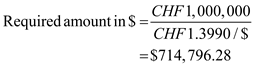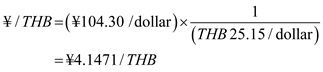## Quiz 2 : The Foreign Exchange MarketLooking for Finance Homework Help?# Quiz 2 : The Foreign Exchange Market

Given information • The Bank's Bid rate; this is the price at which bank is willing to buy \$1 in exchange of CHF 1.3990. • The Bank's ask price; this is the price at which bank is willing to sell dollars in return for CHF1.4000. Calculate required amount in dollars to buy CHF 1,000,000. Step1: M. Inc. must sell dollars to the bank to get the Swiss Francs ( CHF ) in order to make payments to its Swiss Chocolate Supplier. Step 2: Determine dollar cost of the CHF 1,000,000 by dividing the required CHF 1,000,000 with CHF 1.3990 per dollar exchange rate as follows:Thus, the \$714,796.28 is the dollar cost of CHF 1,000,000.

The exchange rate is the rate at which the currencies of different countries are exchanged on a platform called Foreign exchange market. Instances include U.S dollar price of the Yen, the Thai Baht price of the Euro.The exchange rates can be quoted in two ways either direct or indirect. In the context of direct quotes domestic currency price of the foreign exchange in the United States. In the context of indirect quotes the foreign currency price of the domestic currency in the United States.

Given information • Exchange rate of Japanese Yen per dollar ¥104.30/\$ • Thai Baht that are required to purchase a dollar is 25.15 Calculate yen price of Thai baht. Here neither the Japanese yen nor the Thai Baht is the official currency, so in order to determine the Japanese yen price of the Thai Baht calculate cross-rate as follows:Thus, the yen price of baht is ¥4.1471/ THB.

There is no answer for this question

There is no answer for this question